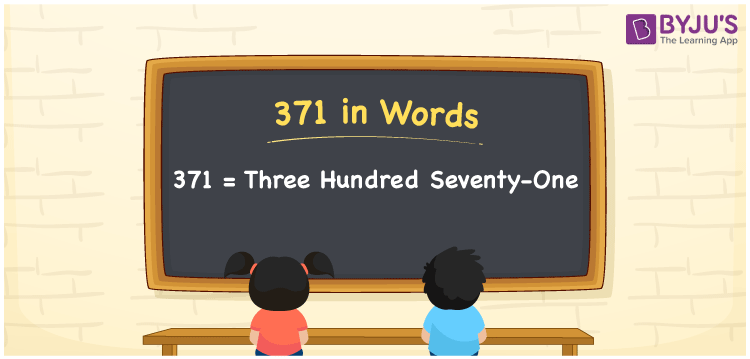# 371 in Words

371 in words is written as Three hundred seventy-one. In both the International System of Numerals and the Indian System of Numerals, 371 is written as Three hundred seventy-one. The number 371 is a Cardinal Number as it represents some quantity. For example, the “cost of the t-shirt is 371 rupees”.

 371 in Words Three hundred seventy-one Three hundred seventy-one in Number 371

## 371 in English Words

We write 371 in English Words using the letters of the English alphabet. Therefore, we read 371 in English as “Three hundred seventy-one”.## How to Write 371 in Words?

To write 371 in words, we shall use the place value chart. In the place value chart, write 3 in the hundreds, 7 in the tens, and 1 in the ones, respectively. Now let us make a place value chart to write the number 371 in words.

 Hundreds Tens Ones 3 7 1

Thus, we can write the expanded form as

3 × Hundred + 7 × Ten + 1 × One

= 3 × 100 + 7 × 10 + 1 × 1

= 300 + 70 + 1

= 371

= Three hundred seventy-one.

371 is a natural number, the successor of 370 and the predecessor of 372.

371 in words – Three hundred seventy-one

• Is 371 an odd number? – Yes
• Is 371 an even number? – No
• Is 371 a perfect square number? – No
• Is 371 a perfect cube number? – No
• Is 371 a prime number? – No
• Is 371 a composite number? – Yes

## Frequently Asked Questions on 371 in Words

### How to write 371 in words?

371 in words is written as Three hundred seventy-one.

### How to write 371 in the International and Indian System of Numerals?

In both, the system of numerals, 371 in words, is written as Three hundred seventy-one.

### What is the successor of 371?

The successor of 371 is 372.